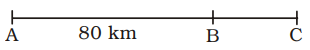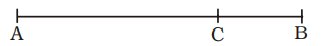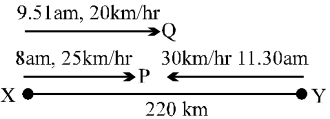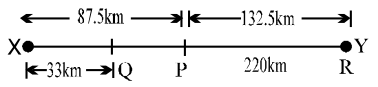## Speed, Time and Distance

#### Speed, Time and Distance

1. Two places A and B are 80 km apart from each other on a highway. A car starts from A and another from B at the same time. If they move in the same direction, they meet each other in 8 hours. If they move in opposite directions towards each other, they meet in 1 hour 20 minutes. Determine the speed of the faster car.
1. 20 kmph
2. 25 kmph
3. 35 kmph
4. 30 kmph

1. Case : I
When the cars are moving in the same direction.Let A and B be two places and C be the place of meeting.
Let the speed of car starting from A be x kmph, and that of car starting from B be y kmph.
Relative speed = (x – y) kmph
According to the question.
(x – y) × 8 = 80
⇒  x – y = 10     ...(i)
Case : II
When the cars are moving in the opposite directions and they meet at point C.Relative speed = (x + y) kmph
Time taken = 1 hour 20 minutes

 = 1 + 1 = 4 hours 3 3

 ∴  (x + y) × 4 = 80 3

 ⇒  x + y = 80 × 3 4

⇒  x + y = 60     ...(ii)
2x = 70
⇒  x = 35
From equation (ii),
x + y = 60
⇒  35 + y = 60
⇒  y = 60 – 35 = 25
∴  Speed of the faster car = 35 kmph

##### Correct Option: C

Case : I
When the cars are moving in the same direction.Let A and B be two places and C be the place of meeting.
Let the speed of car starting from A be x kmph, and that of car starting from B be y kmph.
Relative speed = (x – y) kmph
According to the question.
(x – y) × 8 = 80
⇒  x – y = 10     ...(i)
Case : II
When the cars are moving in the opposite directions and they meet at point C.Relative speed = (x + y) kmph
Time taken = 1 hour 20 minutes

 = 1 + 1 = 4 hours 3 3

 ∴  (x + y) × 4 = 80 3

 ⇒  x + y = 80 × 3 4

⇒  x + y = 60     ...(ii)
2x = 70
⇒  x = 35
From equation (ii),
x + y = 60
⇒  35 + y = 60
⇒  y = 60 – 35 = 25
∴  Speed of the faster car = 35 kmph

1. In a 200 metre race, A beats B by 20 metres; while in a 100 metres race, B beats C by 5
metres. Assuming that the speed of A, B and C remain the same in various races, by how many metres will A beat C in one kilometre race ?
1. 140 metre
2. 145 metre
3. 135 metre
4. 125 metre

1. According to the question,
when A covers the distance of 200 metres, B covers only 200– 20 = 180 metres
Again, in 100 metre race, B beats C by 5 metres.
Hence, if B runs 100 metres, C runs 100–5 = 95 metres
∵  If B runs 100 m, C runs = 95 m
∴  If B runs 180 m, C runs

 = 95 × 180 = 171 m 100

∴  A : B : C = 200 : 180 : 171
Hence, A will beat C by
= 200–171 = 29 m in 200 m race.
i.e., 29 × 5 = 145 m in 1 km race.

##### Correct Option: C

According to the question,
when A covers the distance of 200 metres, B covers only 200– 20 = 180 metres
Again, in 100 metre race, B beats C by 5 metres.
Hence, if B runs 100 metres, C runs 100–5 = 95 metres
∵  If B runs 100 m, C runs = 95 m
∴  If B runs 180 m, C runs

 = 95 × 180 = 171 m 100

∴  A : B : C = 200 : 180 : 171
Hence, A will beat C by
= 200–171 = 29 m in 200 m race.
i.e., 29 × 5 = 145 m in 1 km race.

1. A steamer goes downstream from one port to another in 4 hours. It covers the same distance upstream in 5 hours. If the speed of the stream be 2 km/hr, find the distance between the two ports.
1. 60 km.
2. 45 km.
3. 80 km.
4. 65 km.

1. Let the speed of steamer in still water = x kmph
∴  Rate downstream = (x + 2) kmph
Rate upstream = (x – 2) kmph
Obviously, distance covered downstream and upstream are equal
⇒  4 (x + 2) = 5 (x – 2)
⇒  4x + 8 = 5x – 10
⇒  5x – 4x = 10 + 8 ⇒ x = 18
∴  Rate downstream
= 18 + 2 = 20 kmph
Therefore, the required distance
= Speed downstream × Time
= 20 × 4 = 80 km.

##### Correct Option: C

Let the speed of steamer in still water = x kmph
∴  Rate downstream = (x + 2) kmph
Rate upstream = (x – 2) kmph
Obviously, distance covered downstream and upstream are equal
⇒  4 (x + 2) = 5 (x – 2)
⇒  4x + 8 = 5x – 10
⇒  5x – 4x = 10 + 8 ⇒ x = 18
∴  Rate downstream
= 18 + 2 = 20 kmph
Therefore, the required distance
= Speed downstream × Time
= 20 × 4 = 80 km.

1. A person travels a certain distance on a bicycle at a certain speed. Had he moved 3 km/hour faster, he would have taken 40 minutes less. Had he moved 2km/hour slower, he would have taken 40 minutes more. Find the distance.
1. 45 km.
2. 40 km.
3. 50 km.
4. 55 km.

1. Let the original speed of the person be x km/hr. and the distance be y km.
Case : I

 y − y = 40 minutes x x + 3

 or, 40 hr. 60

 or, y − y = 40 = 2 x x + 3 60 3

 or,   y1 − 1= 1 x (x + 3) 3

 or,   yx + 3 − x= 2 x (x + 3) 3

 or, 3y = 2 x (x + 3) 3

or,   2 x (x + 3) = 9y       ...(i)
Case : II
 = y − y = 40 x − 2 x 60

 or,   y1 + 1+ 2 x − 2 x 3

 or,   yx − x + 2= 2 x (x − 2) 3

 or, 2y = 2 x (x − 2) 3

or, x (x – 2) = 3y       ... (ii)
On dividing equation (i) by (ii) we have,
 2x(x + 3) = 9y x (x − 2) 3y

 or, 2(x + 3) = 3 (x − 2)

or,   2x + 6 = 3x – 6
or,   3x – 2x = 6 + 6 = 12
or,   x = 12 km/hr.
∴  Original speed of the person
= 12 km/hr.
Putting the value of x in equation (ii)
12 (12 – 2) = 3y
or,   3y = 12 × 10
 or,   y = 12 × 10 = 40 3

∴  The required distance = 40km.

##### Correct Option: B

Let the original speed of the person be x km/hr. and the distance be y km.
Case : I

 y − y = 40 minutes x x + 3

 or, 40 hr. 60

 or, y − y = 40 = 2 x x + 3 60 3

 or,   y1 − 1= 1 x (x + 3) 3

 or,   yx + 3 − x= 2 x (x + 3) 3

 or, 3y = 2 x (x + 3) 3

or,   2 x (x + 3) = 9y       ...(i)
Case : II
 = y − y = 40 x − 2 x 60

 or,   y1 + 1+ 2 x − 2 x 3

 or,   yx − x + 2= 2 x (x − 2) 3

 or, 2y = 2 x (x − 2) 3

or, x (x – 2) = 3y       ... (ii)
On dividing equation (i) by (ii) we have,
 2x(x + 3) = 9y x (x − 2) 3y

 or, 2(x + 3) = 3 (x − 2)

or,   2x + 6 = 3x – 6
or,   3x – 2x = 6 + 6 = 12
or,   x = 12 km/hr.
∴  Original speed of the person
= 12 km/hr.
Putting the value of x in equation (ii)
12 (12 – 2) = 3y
or,   3y = 12 × 10
 or,   y = 12 × 10 = 40 3

∴  The required distance = 40km.

1. Distance between two stations X and Y is 220 km. Trains P and Q leave station X at 8 a.m. and 9.51 a.m. respectively at the speed of 25 kmph and 20 kmph respectively for journey towards Y. A train R leaves station Y at 11.30 a.m. at a speed of a 30 kmph. for journey towards X. When will P be at equal distance from Q and R ?
1. 12:48 pm.
2. 12:30 pm.
3. 12:45 pm.
4. 11:48 pm.

1.Distance covered by P till 11.30 a.m.
= (11.30 a.m. – 8 a.m.) × 25km

 = 3 1 × 25 = 87.5 km. 2

Distance covered by Q till 11.30 a.m.
= (11.30 – 9.51 am) × 20
 = 1 39 hrs. × 20 = 33 km 60

So, at 11.30 a.m. the three trains will be at positions shown below :P gains 5 km every hour over Q.
Relative speed of P w.r.t. R = 20 + 30 = 50 km per hr
Let P be at equal distance from Q and R after t hours.
∴  (87.5 – 33) + 5t
= 132.5 –55t
or,   54.5 + 5t = 132.5 – 55t
or,   60 t = 78
 or,   t = 78 hrs. 60

= 1 hr 18 minutes
11.30 am + 1 hr. 18 min.
= 12.48 pm
At 12.48 pm, P would have covered a distance
= (12.48 pm – 8 am) × 25
= 120 km
Therefore, P will be at equal distance from Q and R at 12.48 pm

##### Correct Option: ADistance covered by P till 11.30 a.m.
= (11.30 a.m. – 8 a.m.) × 25km

 = 3 1 × 25 = 87.5 km. 2

Distance covered by Q till 11.30 a.m.
= (11.30 – 9.51 am) × 20
 = 1 39 hrs. × 20 = 33 km 60

So, at 11.30 a.m. the three trains will be at positions shown below :P gains 5 km every hour over Q.
Relative speed of P w.r.t. R = 20 + 30 = 50 km per hr
Let P be at equal distance from Q and R after t hours.
∴  (87.5 – 33) + 5t
= 132.5 –55t
or,   54.5 + 5t = 132.5 – 55t
or,   60 t = 78
 or,   t = 78 hrs. 60

= 1 hr 18 minutes
11.30 am + 1 hr. 18 min.
= 12.48 pm
At 12.48 pm, P would have covered a distance
= (12.48 pm – 8 am) × 25
= 120 km
Therefore, P will be at equal distance from Q and R at 12.48 pm